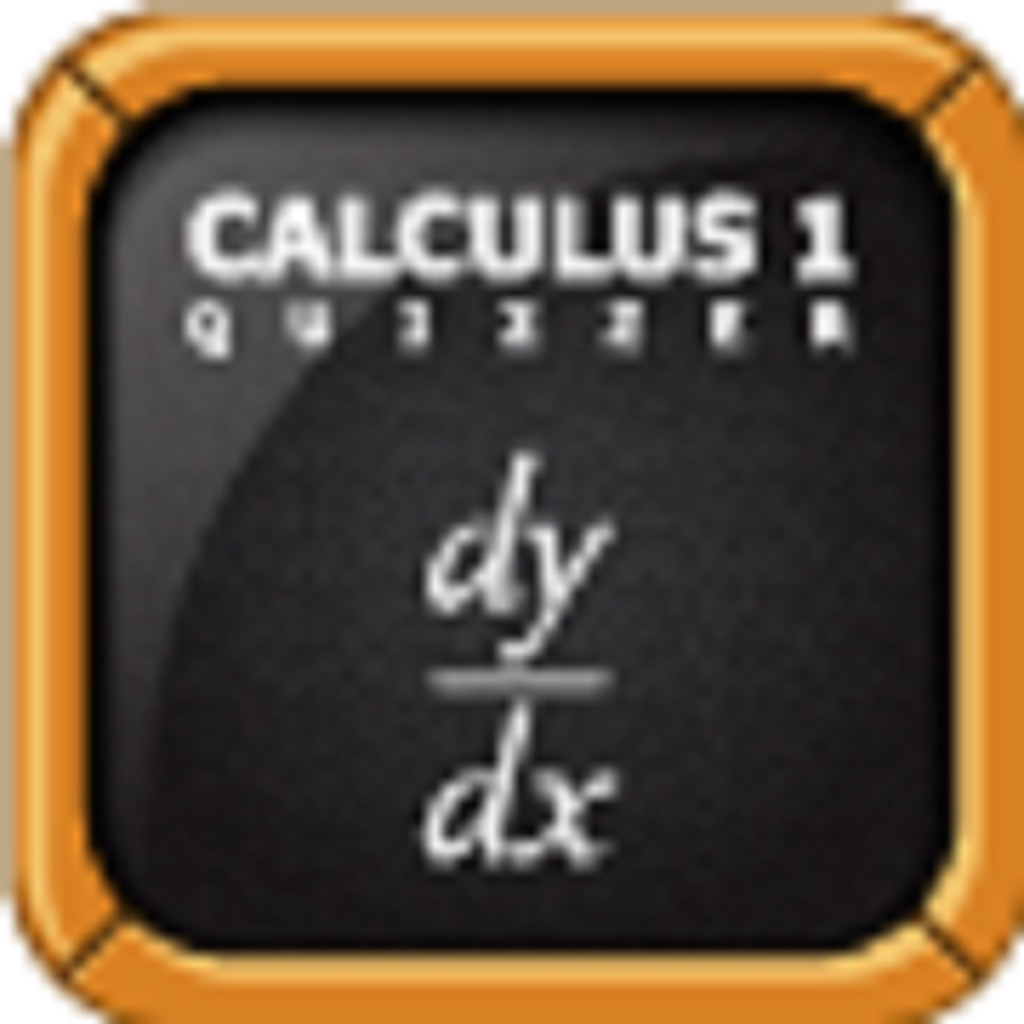Calculus 1 Quizzer# Calculus 1 Quizzer

\$2.99
0

0 Ratings

Release Date

2018-09-20

Size

4.3 MB

### Screenshots for iPhone

iPhone
Calculus 1 Quizzer Description
Calculus 1 Quizzer is a quiz application for students planning on or currently taking a college or university level Calculus 1 class. The application has 16 different levels. Each time you take a quiz, (even if you stay in the same level), the questions will be different. Each question has a tip to help the user solve the problem. It also has multiple profile selection and score tracking capabilities.

The following are the topics covered in each level:

1. Functions:
Introduction to when an equation is a function (horizontal line test). Includes trigonometric functions and polynomials as a pre-calculus review. Also covers linear functions, inverse functions, even-odd functions, rational functions, exponential functions, logarithms, etc.  Finally some basic concepts such as domain and range of a function and inverses.

2. Limits and Continuity
Limits are usually the first thing covered in calculus, and would be best to be introduced early.  Convergence and divergence are central to this level. Includes limits of polynomials, rational functions, and trigonometric functions.

3. Differentiability and Derivative of a Function
The definition of derivative  ( lim h->o of [f(x+h)-f(x)]/h ) depends on limits and it is best that students understand limits of functions first. This level covers the concept of a derivative as the slope of a function, basic properties of taking limits, tangent and normal lines, and how to calculate a derrivative using the lim h->0 method.

4. Power Rule, Product Rule and Quotient Rule
This level simplifies the computation of derivatives using the above rules.  It also covers the derrivatives of powers, polynomials, and rational functions.

5. Derivatives by the Chain Rule
This level covers the derivatives of composite functions. It also covers derivatives of logarithms and exponential functions.

6. Derivative of the trigonometric functions
This level covers the derivatives of Sine, Cosine, tangent, cotangent, secant, and cosecant.  Also allows for practice of the previously learned rules.

7. Inverse Functions and their derivatives
This level reviews when a function is invertible (horizontal line test).

8. Second Derivatives, concavity, and physical applications
This level introduces the notion of a second derivative and covers the concept of concavity.

9. Graphs of functions and curve Sketching
This level covers extreme value theorem, mean value theorem, relative max and mins by first and second derrivative tests, inflection points, vertical and horizontal asymptotes, increasing/decreasing intervals, concave up and concave down.

10. Implicit Differentiation
This level covers the concepts of taking the derivative of an ellipse, hyperbola, circle, and other things that are not defined as functions but rather are defined by implicit equations (such as x^2 + y^2 = 1 for the circle).

11. Related Rates and Optimization
This level focuses on the applications of implicit differentiation.  It also reviews simple geometric formulas such as area of a circle, sphere, triangle, cone, etc.

12. Linear Approximation and differentials.
This should be introduced by the standard definition f(x+a) = f(a) + f'(a)(x-a). This level also covers mean value theorem and intermediate value theorem.

13. Area under a curve and Riemann sums
This level covers the concept of left, middle, and right Riemann sums with a finite number of rectangles.

14. Anti-derivatives and integration
This level covers the Fundamental Theorem of Calculus and the relation of definite integrals and area under a curve. Basic properties of integration are also covered.

15. Integration by Substitution Method
This level covers how to do u-substitution and when it is necesary (sometimes refered to as the inverse-chain rule), as well as integration.

16. Solid of a revolution
This level covers the concept of a solid of a revolution as well as how to compute it's volume through disc/washer method and cylindrical shell method.
Calculus 1 Quizzer 2.1 Update
2018-09-20 Version History
This version downgrades the iOS requirements from 10 to 9.
More
Price:
\$2.99
Version:
2.1
Size:
4.3 MB
Release Date:
2018-09-20
Developer: